# 1. 梯度爆炸问题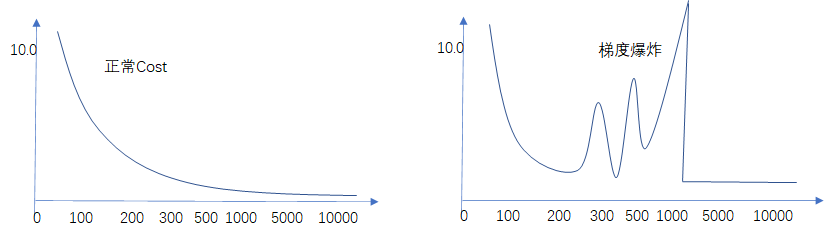cost:  0.00532
......
cost:  1097.2125
cost:  nan
cost:  nan


        #更换默认tanh激活函数
cell_list = tf.contrib.rnn.BasicLSTMCell(self.cell_size,
forget_bias=1.0,
state_is_tuple=True,
activation=tf.nn.relu)


    def _weight_variable(self, shape, name='weights'):
initializer = tf.random_normal_initializer(mean=0., stddev=1.0,)
return tf.get_variable(shape=shape, initializer=initializer, name=name)

def _bias_variable(self, shape, name='biases'):
initializer = tf.constant_initializer(0.1)
return tf.get_variable(name=name, shape=shape, initializer=initializer)


# 2. 解决方案

## 2.1. 换回tanh激活函数？

r e l u ( x ) = m a x ( x , 0 ) = { x , x ⩾ 0 0 x < 0 relu(x)=max(x,0)= \left\{\begin{matrix} x, & x\geqslant 0\\ 0 & x<0 \end{matrix}\right.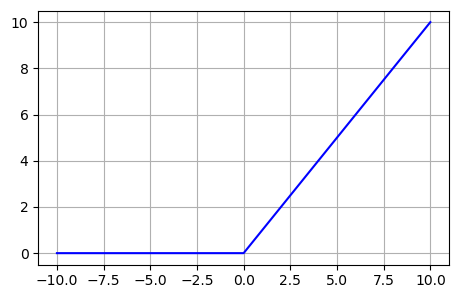• 没有饱和区，不存在梯度消失问题。
• 没有复杂的指数运算，计算简单、效率提高。
• 实际收敛速度较快，比 Sigmoid/tanh 快很多。
• 比 Sigmoid 更符合生物学神经激活机制。

Relu的缺点：

• 在训练的时候，ReLU单元比较脆弱并且可能“死掉”。举例来说，当一个很大的梯度，流过ReLU的神经元的时候，可能会导致梯度更新到一种特别的状态，在这种状态下神经元将无法被其他任何数据点再次激活。如果这种情况发生，那么从此所以流过这个神经元的梯度将都变成0。也就是说，这个ReLU单元在训练中将不可逆转的死亡，因为这导致了数据多样化的丢失。

• 如果学习率设置得太高，可能会发现网络中40%的神经元都会死掉（在整个训练集中这些神经元都不会被激活）。通过合理设置学习率，这种情况的发生概率会降低。

t a n h ( x ) = 2 1 + e − 2 x − 1 tanh(x) = \frac{2} {1+e^{-2x}}-1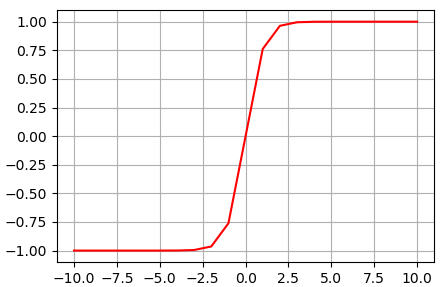sigmoid和tanh：

• sigmoid在输入处于[-1,1]之间时，函数值变化敏感，一旦接近或者超出区间就失去敏感性，处于饱和状态，影响神经网络预测的精度值；
• tanh的变化敏感区间较宽，导数值渐进于0、1，符合人脑神经饱和的规律，比sigmoid函数延迟了饱和期；
• tanh在原点附近与y=x函数形式相近，当激活值较低时，可以直接进行矩阵运算，训练相对容易；
• tanh和sigmoid都是全部激活（fire），使得神经网络较重（heavy）。

relu时比tanh收敛更快，且准确率更高

## 2.2. 优化初始化权重

    def _weight_variable(self, shape, name='weights'):
initializer = tf.random_normal_initializer(mean=0., stddev=0.1,)
return tf.get_variable(shape=shape, initializer=initializer, name=name)

def _bias_variable(self, shape, name='biases'):
initializer = tf.constant_initializer(0.01)
return tf.get_variable(name=name, shape=shape, initializer=initializer)


tf.random_normal_initializer(mean=0.0, stddev=0.1, seed=3)

• mean：正态分布的均值，默认值 0，一个 python 标量或一个标量张量.要生成的随机值的均值.
• stddev：正态分布的标准差， 默认值 1，一个 python 标量或一个标量张量.要生成的随机值的标准偏差.
• seed：随机种子，指定seed的值相同生成同样的数据，一个 Python 整数.用于创建随机种子.查看 tf.set_random_seed 行为.
• dtype：数据类型,只支持浮点类型

import matplotlib.pyplot as plt
import math
import numpy as np

def testInitWeight():
x = np.random.uniform(0,1,30*25)
t = 10000
z_lst = np.empty(t)
mu = [0,0,0]
sigma = [1.0,0.1,0.01]
for j in range(3):
for i in range(t):
w = np.random.normal(mu[j], sigma[j], 30*25)
b = 0
# z为加权和
z = np.sum(x * w) + b
z_lst[i] = z

print ('z 均值：', np.mean(z_lst))
print ('z 方差：', np.var(z_lst))
plt.subplot(1,3,(j+1))
plt.grid()

plt.hist(z_lst, bins=10)

plt.show()

if __name__ == '__main__':
testInitWeight()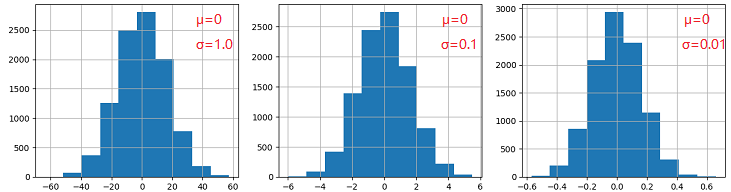通过实践，标准差设置小些，如上所示“stddev=0.1”，训练过程中较稳定，减少了梯度爆炸的发生。

## 2.3. 梯度修剪

Tensorflow梯度修剪函数为tf.clip_by_value(A, min, max)：

    def compute_cost(self):
losses = tf.contrib.legacy_seq2seq.sequence_loss_by_example(
[tf.reshape(self.pred, [-1], name='reshape_pred')],
[tf.reshape(self.ys, [-1], name='reshape_target')],
[tf.ones([self.batch_size * self.n_steps*self.output_size], dtype=tf.float32)],
average_across_timesteps=True,
softmax_loss_function=self.ms_error,
name='losses'
)

with tf.name_scope('average_cost'):
self.cost = tf.div(
tf.reduce_sum(losses, name='losses_sum'),
self.batch_size_,
name='average_cost')
tf.summary.scalar('cost', self.cost)

def train_optimizer(self):
# 计算导数，cost为损失函数
# 限定导数值域-1到1
# 将处理后的导数继续应用到LSTM算法中


# 3. 原理说明

## 3.1. 什么是梯度爆炸

• 模型无法从训练数据中获得更新（如低损失）。
• 模型不稳定，导致更新过程中的损失出现显著变化。
• 训练过程中，模型损失变成 NaN。

RNN结果出现nan值？梯度爆炸，导致结果不收敛。都是梯度太大惹的祸，所以可以通过减小学习率（梯度变化直接变小）、减小batch size（累积梯度更小）、 features规格化（避免突然来一个大的输入）。

## 3.2. 链式法则

x x 是实数， f f g g 是从实数映射到实数的函数。假设 y = g ( x ) y=g(x) ，并且 z = f ( g ( x ) ) = f ( y ) z=f(g(x))=f(y) 。那么链式法则是说：

d z d x = d z d y d y d x \frac {dz}{dx}=\frac {dz}{dy}\frac {dy}{dx}

d z d x = f ′ ( g ( x ) ) g ′ ( x ) \frac {dz}{dx}=f'(g(x))g'(x)

Hinton在它的IRNN论文里面（arxiv：[1504.00941] A Simple Way to Initialize Recurrent Networks of Rectified Linear Units）是很明确的提到的:

f ( x ) = x f(x)=x
n e t t = U x t + W ( U x t − 1 + W h t − 2 ) net_{t} = Ux_{t} + W(Ux_{t-1}+Wh_{t-2})

n e t t n e t t 1 = W n \frac{net_{t}}{net_{t1}}=W^{n}

## 3.3. 梯度修剪原理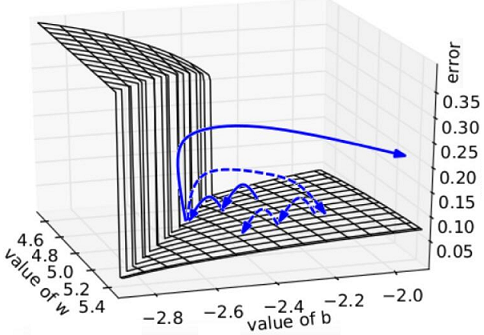## 3.4. 权重正则化

​ 如果用一句话解释：正则化就是通过增加权重惩罚(penalty)项到损失函数，让网络倾向于学习小一点的权重，从而达到抑制过拟合，增加模型泛化能力的效果。常见的正则化方法有 L 1 L1 正则化， L 2 L2 正则化和Dropout正则化等。其中， L 2 L2 正则化的公式：

L = L 0 + λ 2 ∑ i = 1 n ( w 2 ) L = L_{0} + \frac{ \lambda}{2} \sum_{i=1}^{n}(w^{2})

• tf.get_collection 从特定集合中取出对应的资源

# 4. 小结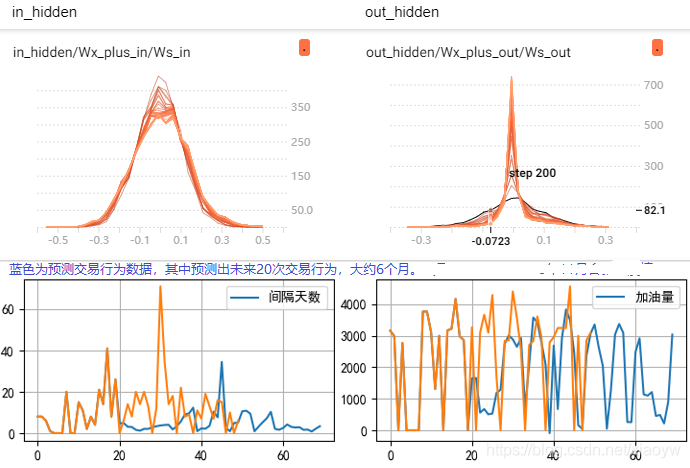《深度学习–解决梯度爆炸方法（含TensorFlow代码）》 CSDN博客， 超屌的温jay ，2018年6月
《激活函数 sigmoid、tanh、relu》 简书 ， SpikeKing ，2019年1月
《Tensorflow LSTM实现多维输入输出预测实践详解》 CSDN博客 ，肖永威 ，2021年3月
《基于tensorflow的正则化实现》 简书 ， AlexChung16 ，2020年5月

08-2003-171951
01-06524
04-2437
02-18784
12-201万+
06-08412
12-243316
06-261万+
06-181143
08-08886
04-24666
07-081万+
12-081902
10-181万+
01-05366
12-12209
05-095770
04-01775